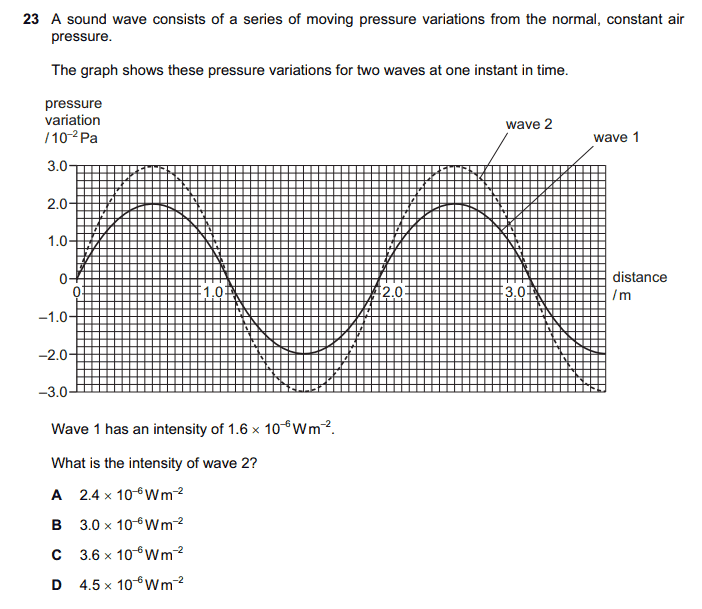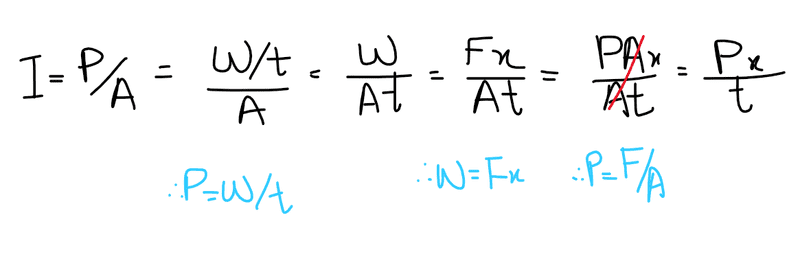# Intensity of two sound pressure waves

Homework Statement
Ans: C

I tried to use the intensity formulae and after manipulating it got an expression which gave me wrong answer.
It seems the areas are not equivalent somehow, any explanation?
Relevant Equations
I = P/AHi @iQadmat and welcome to PF.

How does the intensity depend on the amplitude? You can read the amplitudes of the two waves off the graph and compare.

Hi @iQadmat and welcome to PF.

How does the intensity depend on the amplitude? You can read the amplitudes of the two waves off the graph and compare.
Hi and thanks for welcoming me!

I know the intensity is directly proportional to amplitude^2 frequency^2 and inversely proportional to radius^2, but my point was the areas (which I showed in ss) are different somehow and I want to understand the differences between them and what they represent.

So far my understanding*
[The initial 'A' is showing the area at which the power from source is acting upon but the final 'A' (later derived from 'F') is not the initial 'A' but is the area of the wave particle which times by pressure of wave equals 'F' .]

•Delta2
The areas are irrelevant. This is a comparison of intensities of two different sources at the same point in space and time. If you must, assume that the two sources are at the same distance from two detectors with identical areas. Compare the detectors' readings. Clearly, wave 2 has greater intensity than wave 1. By what factor?

The areas are irrelevant. This is a comparison of intensities of two different sources at the same point in space and time. If you must, assume that the two sources are at the same distance from two detectors with identical areas. Compare the detectors' readings. Clearly, wave 2 has greater intensity than wave 1. By what factor?
I got it now, Thanks for your help!

•Delta2 and kuruman